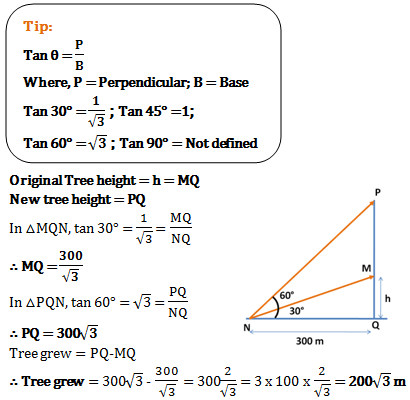# Mensuration and Trigonometry - Aptitude Questions and Answers - Set 1

1)   A triangular ground has 8km, 10km and 6km as its sides. It is to be painted at the rate of Rs. 8 per sq.km. How much will it cost?

a. Rs. 256
b. Rs. 3840
c. Rs. 384
d. Rs. 192
 Answer  Explanation ANSWER: Rs. 192 Explanation: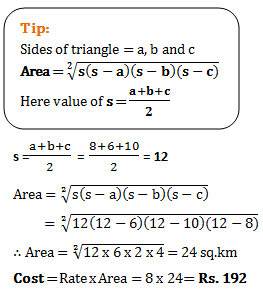2)   Find perimeter of △LMN, if midpoints of its sides are joined to form △PQR.  Also midpoints of △PQR are joined to get △CDE and CD=3, DE=8 and CE=4.

a. 30
b. 72
c. 60
d. 90
 Answer  Explanation ANSWER: 60 Explanation: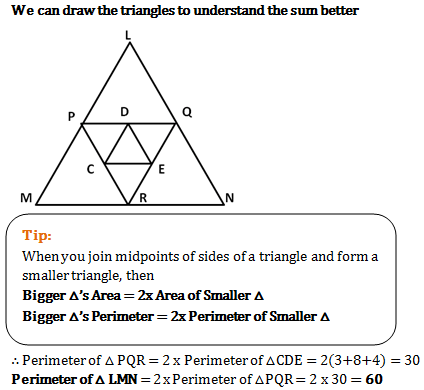3)   Length of a rectangular ground is 15m more than its breadth. What will be its area if Length : Breadth is 7:4?

a. 700 sq.m.
b. 450 sq.m.
c. 900 sq.m.
d. 850 sq.m.
 Answer  Explanation ANSWER: 700 sq.m. Explanation: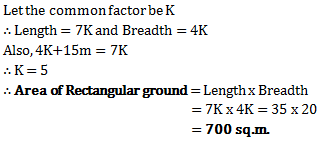4)   A rectangular margin has a length of 48cm and a total area of 840 sq.cm. The area becomes 2(6/7) times the initial area by just increasing the breadth. How much is the perimeter of the

a. 1.69 m
b. 1.85 m
c. 1.96 m
d. 2.25 m
 Answer  Explanation ANSWER: 1.96 m Explanation: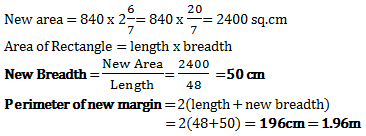5)   A plot has a concrete path within its borders on all sides having uniform width of 4m. The plot is rectangular with sides 20m and 15m. Charge of removing concrete is Rs. 6 per sq.m. How much is spent in removing all the concrete?

a. Rs. 1548
b. Rs. 1296
c. Rs. 1500
d. Rs. 1083
 Answer  Explanation ANSWER: Rs. 1296 Explanation: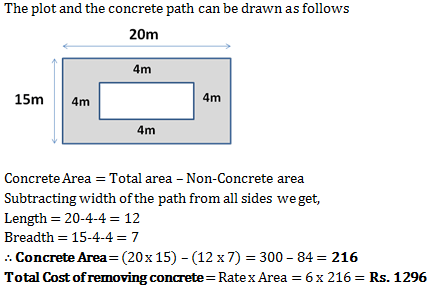6)   If side of each square is 1cm, then shaded area =? Sq.cm.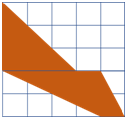a. 11 sq.cm.
b. 8 sq.cm.
c. 12.5 sq.cm
d. 9.5 sq.cm.
 Answer  Explanation ANSWER: 9.5 sq.cm. Explanation: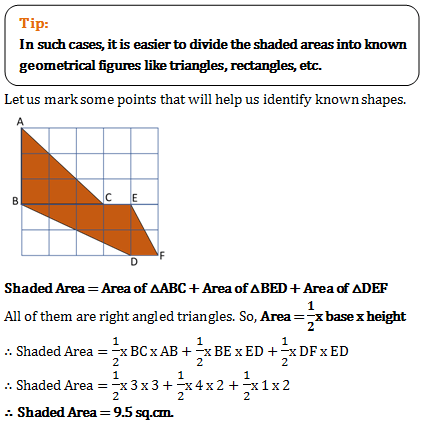7)   What will be maximum possible length of a pole in a room with dimensions 10 cm x 12 cm x 8 cm?

a. 10 cm
b. 1411cm
c. 277cm
d. 12 cm
 Answer  Explanation ANSWER: 277cm Explanation: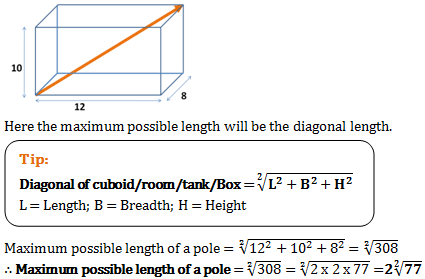8)   A 4080 cu.cm cubical room can contain how many maximum number of boxes having dimensions 4 cm, 3 cm and 2 cm?

a. 170
b. 185
c. 160
d. 155
 Answer  Explanation ANSWER: 170 Explanation: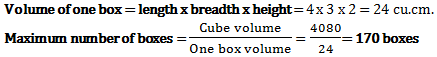9)   A rectangular wall has its length, breadth and height in the ratio 6:5:3. What is its breadth if entire surface area is 504 sq.m?

a. 53 sq.m
b. 23 sq.m
c. 22 sq.m
d. 102 sq.m
 Answer  Explanation ANSWER: 102 sq.m Explanation: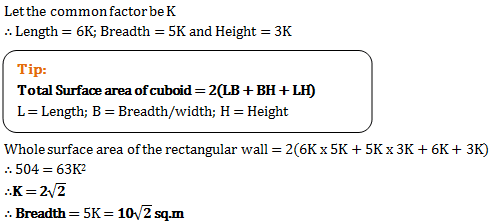10)   On decreasing each side of cube by 21%, its surface area decreases by?

a. 38.47%
b. 37.59%
c. 38.95%
d. -33.33%
 Answer  Explanation ANSWER: 37.59% Explanation: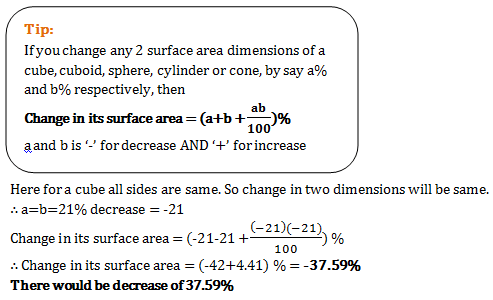11)   2 cylinders have their radii into ratio 4:7. If their heights are in the ration of 21:8 then their volumes would be in what ratio?

a. 2:3
b. 7:6
c. 6:7
d. 3:2
 Answer  Explanation ANSWER: 6:7 Explanation: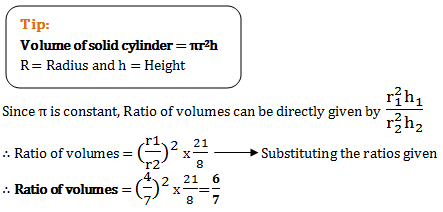12)   What will be total cost of polishing curved surface of a wooden cylinder at rate of Rs. 20 per sq.m, if it has 40 cm diameter and 7m height?

a. Rs. 480
b. Rs. 384
c. Rs. 352
d. Rs. 176
 Answer  Explanation ANSWER: Rs. 176 Explanation: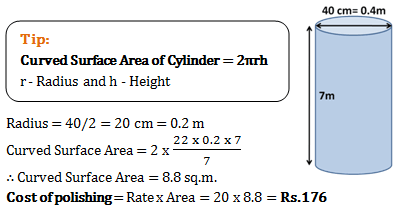13)   Mohan looks at a tree top and the angle made is 45°. He moves 10 cm back and again looks at the tree top but this time angle made is 30°.  How high is the tree top from ground?

a. 10/(3+1) cm
b. 203 cm
c. 20 cm
d. 10/(3-1) cm
 Answer  Explanation ANSWER: 10/(3-1) cm Explanation: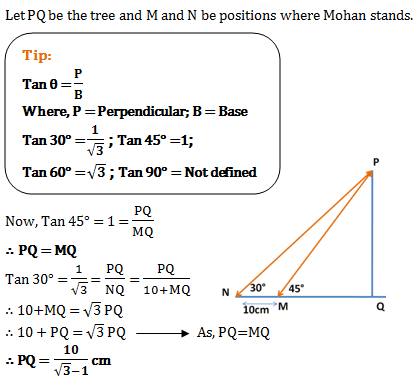14)   There is a tower of 10m between two parallel roads. The angles of depression of the roads from the top of the tower are 30° and 45°.  How far are the roads from each other?

a. 27.32 m
b. 29.56 m
c. 203 m
d. 10/3 m
 Answer  Explanation ANSWER: 27.32 m Explanation: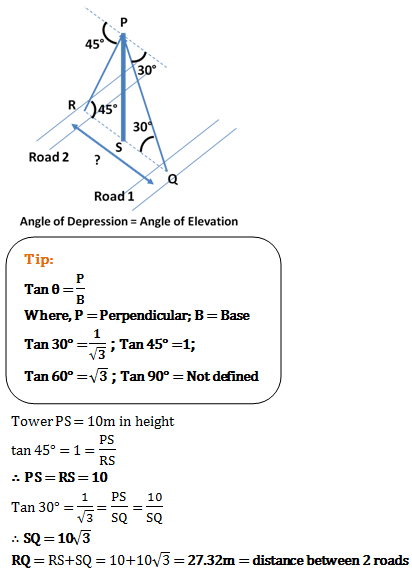15)   A tree breaks and falls to the ground such that its upper part is still partially attached to its stem. At what height did it break, if the original height of the tree was 24 cm and it makes an angle of 30° with the ground?

a. 12 cm
b. 8 cm
c. 9.5 cm
d. 7.5 cm
 Answer  Explanation ANSWER: 8 cm Explanation: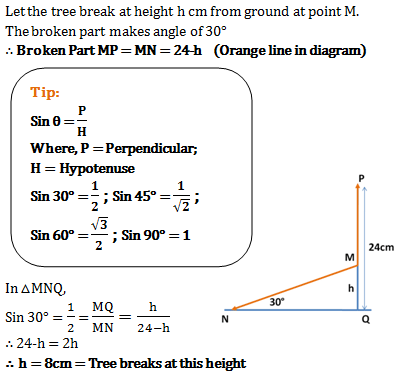16)   Shadow of a man is 1/3 times the height of the man. What will be the sun’s angle of elevation?

a. 135°
b. 45°
c. 30°
d. 60°
 Answer  Explanation ANSWER: 60° Explanation: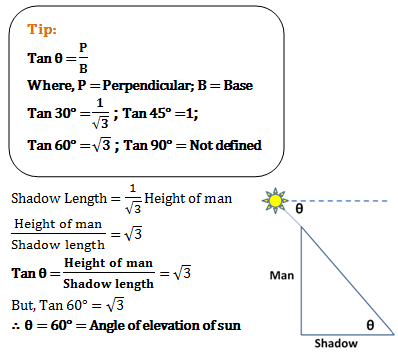17)   Due to sun, a 6ft man casts a shadow of 4ft, whereas a pole next to the man casts a shadow of 36ft. What is the height of the pole?

a. 63 ft
b. 72 ft
c. 54 ft
d. 48 ft
 Answer  Explanation ANSWER: 54 ft Explanation: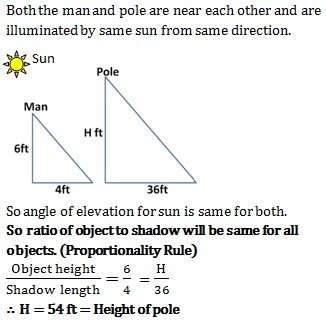18)   Tree top’s angle of elevation is 30° from a point on ground, 300m away the tree. When the tree grew up its angle of elevation became 60° from the same point. How much did the tree grow?

a. 1003 m
b. 2003 m
c. 300(1/3)
d. 200/3 m
 Answer  Explanation ANSWER: 2003 m Explanation: